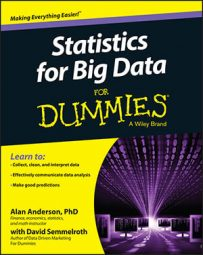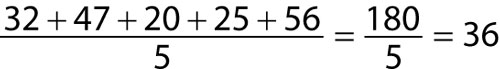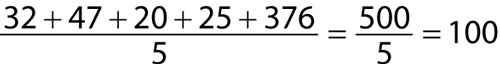##### Statistics for Big Data For DummiesA statistic is said to be robust if it isn’t strongly influenced by the presence of outliers. For example, the mean is not robust because it can be strongly affected by the presence of outliers. On the other hand, the median is robust — it isn’t affected by outliers.

For example, suppose the following data represents a sample of household incomes in a small town (measured in thousands of dollars per year):

32, 47, 20, 25, 56

You compute the sample mean as the sum of the five observations divided by five:The sample mean is \$36,000 per year. Most of the households in the sample are very close to this value.

Suppose instead that the sample consists of the following values:

32, 47, 20, 25, 376

Because the household income of \$376,000 is substantially greater than the next closest household income of \$32,000, the household income of \$376,000 can be considered to be an outlier.

With the outlier, the sample mean is now as follows:This measure isn’t representative of most of the households in the town. Thus, the usefulness of the mean is compromised in the presence of outliers.

You compute the median of the sample by sorting the data from lowest to highest and then finding the value which divides the sample in half. In other words, half of the observations are below the median, and half are above.

The first sample:

32, 47, 20, 25, 56

The sorted sample:

20, 25, 32, 47, 56

In this case, the median is 32 because half of the remaining observations are below 32 and half are above it.

The second sample:

32, 47, 20, 25, 376

The sorted sample:

20, 25, 32, 47, 376

Despite the presence of the outlier of 376, the median is still 32. It hasn’t been affected by the outlier. This shows that unlike the mean, the median is robust with respect to outliers.

Other examples of robust statistics include the median, absolute deviation, and the interquartile range.